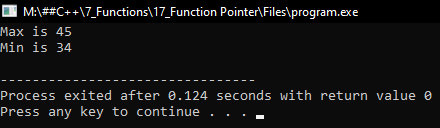# Function Pointer in C++

## Function Pointer in C++ with Examples:

In this article, I am going to discuss Function Pointer in C++ Language with examples. Please read our previous article, where we discussed Scoping Rule in C++ with examples.

##### Function Pointer in C++:

We know well that we can have a pointer to any data type, any primitive data type, we can have a pointer of type class. Can we have a pointer to a function also? Yes, below we have one function,

void display(){
cout << “Welcome” << endl;
}

The name of the function is ‘display’ and it is not returning anything. Now from the main function if we have to call this function then we can simply write ‘display ()’.

int main(){
display();
}

So ‘Welcome’ will be displayed. Now instead of directly calling the name, can we use a pointer to a function? Let us see.

##### Declaration of Function Pointer in C++:

void (*fp)();

Here we have defined a pointer to a function and we are calling the pointer as ‘fp’ and the return type is void because the display function is of the ‘void’ type. We gave blank parenthesis as the display function has no parameters. So, this is the method of declaring a pointer to a function and when you declared a pointer to a function pointer name with ‘*’ must be inside the brackets otherwise it will not be a pointer to a function. This is a declaration of a pointer.

##### Initialization of Function Pointer in C++:

fp = display;

Here we have assigned the ‘fp’ pointer to the ‘display’ function. So, the address of that function will be stored in this pointer. This is the initialization of the function pointer. Now how to call this? Let us see.

##### Calling the Function with Pointer in C++:

(*fp)();

We have written the pointer name with parenthesis the leave empty parenthesis as the ‘display’ function is not taking any parameter. So this is a function call. For declaration, only in place of function name do we have to write down the pointer. When you are declaring a pointer to a function everything should be the same as the signature of a prototype of a function except the function name. Then for initialization, give the function name directly. And for calling give the function pointer name and give the brackets. In this way, a pointer can be assigned to a function.

##### Example of Function Pointer

Now let us take two functions ‘max’ and ‘min’,

int max(int a, int b){
return a>b?a:b;
}
int min(int a, int b){
return a<b?a:b;
}

‘max’ is for finding the maximum of two integers and ‘min’ is for finding the minimum of two integers. Let us declare the function pointer for the ‘max’ and ‘min’ functions.

int (*fp)(int, int);

As ‘max’ and ‘min’ return types are ‘int’ so here we have taken int and also both the functions are taking two ‘int’ values as parameters so here also we give two ‘int’ inside parenthesis but we haven’t written nicknames of the parameter. Just write only datatype. So, this is the declaration of function pointer.

fp = max;

So ‘fp’ is assigned to ‘max’, now we will call the ‘max’ function as,

(*fp)(10, 5);

This will call the max function with 10 and 5 parameters.

Now if we assigned ‘fp’ to ‘min’ as,

fp = min;

(*fp)(10, 40);

Here min function will be called. Different functions are called because the pointer is pointing to different functions. So, this is the same name but with different functions or different operations. So, this is just like polymorphism. In function overriding, internally, function pointers are used for achieving a runtime polymorphism. This means that one function pointer can point to any function which is having the same signature. Now let us write the full program for the function pointer.

##### Function Pointer Program in C++:
```#include <iostream>
using namespace std;
int max(int a, int b)
{
return a > b ? a : b;
}

int min(int a, int b)
{
return a < b ? a : b;
}

int main()
{
int (*fp) (int, int);
fp = max;
cout << "Max is " << (*fp) (45, 34) << endl;
fp = min;
cout << "Min is " << (*fp) (45, 34) << endl;
}
```
###### Output: